# Abstract

This work presents an application of a recursive estimator of states and parameters in a fed-batch penicillin production process based on the use of the extended Kalman filter. The estimated state variables were the cell, substrate, product and dissolved oxygen concentrations, the fermenter volume and the oxygen transfer coefficient. A simplified model of this process was used for the filter, and the actual values for product amount and concentration of dissolved oxygen with independent random Gaussian white noise were obtained using a deterministic and nonstructured mathematical model. The influence of the filter parameters, initial deviations and presence of noise on the observed variables was analyzed. In addition, estimator performance was verified when the parameters and the structure of the process model were changed. The extended Kalman filter implemented was found to be suitable to predict the states of the system and the model parameters. Therefore, it can be used for optimization and control purposes in a fermentative process which requires some state variables that are measured with a long delay time or unmeasured parameters.

extended Kalman filter; fed-batch bioreactor; penicillin process

STATE ESTIMATION AND PARAMETER IDENTIFICATION IN A FED-BATCH PENICILLIN PRODUCTION PROCESS

J.A.D. RODRIGUES 1* * To whom correspondence should be addressed , M. ZAIAT 2 and R. MACIEL FILHO 3

1Departamento de Engenharia Química e Alimentos - Escola de Engenharia Mauá - IMT, Estrada das Lágrimas 2035, CEP 09.580-900, São Caetano do Sul-SP, Brazil.

2Departamento de Hidráulica e Saneamento - Escola de Engenharia de São Carlos - USP, Av. Carlos Botelho 1465, CEP 13.560-250, São Carlos-SP, Brazil.

3Departamento de Processos Químicos - Faculdade de Engenharia Química - UNICAMP, Caixa Postal 6066, CEP 13.081-970, Campinas-SP, Brazil.

(Received: April 14, 1998; Accepted: February 2, 1999)

Abstract - This work presents an application of a recursive estimator of states and parameters in a fed-batch penicillin production process based on the use of the extended Kalman filter. The estimated state variables were the cell, substrate, product and dissolved oxygen concentrations, the fermenter volume and the oxygen transfer coefficient. A simplified model of this process was used for the filter, and the actual values for product amount and concentration of dissolved oxygen with independent random Gaussian white noise were obtained using a deterministic and nonstructured mathematical model. The influence of the filter parameters, initial deviations and presence of noise on the observed variables was analyzed. In addition, estimator performance was verified when the parameters and the structure of the process model were changed. The extended Kalman filter implemented was found to be suitable to predict the states of the system and the model parameters. Therefore, it can be used for optimization and control purposes in a fermentative process which requires some state variables that are measured with a long delay time or unmeasured parameters.

Keywords: extended Kalman filter, fed-batch bioreactor, penicillin process.

INTRODUCTION

Most fermentative processes are coupled with several instruments that enable the measurement of the state of the system. The incorporation of computers into these processes has increased with the implementation of data acquisition systems (Stephanopoulos and San, 1984).

A great potential use for this hardware is to predict fermenter states and parameters of the process model in order to facilitate the study of performance and stability, mainly for those fermentative processes in which cellular growth is important but measurement of cell concentration presents a long delay time (Engasser, 1988).

Therefore, the recursive state estimator can be used for optimization and control purposes in those batch and fed-batch fermentative processes which present state variables as a function of time, thus making possible the implementation of special operating strategies which optimize objective functions (Shimizu, 1993). In addition, it can be applied to estimate the dissolved oxygen concentration and oxygen transfer coefficient in strict aerobic processes (Lee et al., 1991).

This work presents an evaluation of the quality of a recursive state estimator, based on the extended Kalman filter applied to a fed-batch penicillin production process. First, the influence of filter parameters, initial deviations and the presence of noise on the observed variables was analyzed. Then, estimator performance was observed when the parameters and the structure of the process model were changed.

The main advantage of this investigation was the estimation of some state variables (cell, substrate, penicillin and dissolved oxygen concentrations and fermenter volume) and one parameter (oxygen transfer coefficient), some of which were difficult to measure or to measure with a long delay time, from only two observed variables (amount of penicillin and dissolved oxygen concentration).

THEORETICAL PRINCIPLES

The Fed-Batch Penicillin Production Process Model

The production phase of the penicillin production process was mathematically simulated for a fed-batch fermenter with the addition of substrate. The bioreactor was considered completely stirred and isothermal with a variable agitation system and an aeration system.

The proposed mathematical model was deterministic and nonstructured, based on Contois growth-type kinetics with yield coefficients such as specific rate of growth, specific rate of substrate consumption, specific rate of oxygen uptake and specific rate of production; endogenous and maintenance metabolism, product inhibition due to an excess of substrate and hydrolysis of the penicillin. The volumetric oxygen transfer coefficient was estimated using the modified Reynolds number, the power number and apparent viscosity as a function of cell concentration (Rodrigues and Maciel Filho, 1996).

The material balance, kinetic models, oxygen transfer and volumetric equations are shown bellow. This resulting model was solved by a fourth-order Runge-Kutta-Gill variable step algorithm and the parameters were obtained from experiments and literature (Rodrigues,1996).

Material balance equations:(1)(2)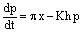(3)(4)(5)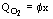(6)

Kinetic equations: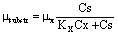(7)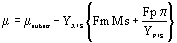(8)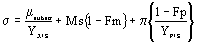(9)(10)(11)(12)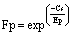(13)

Oxygen transfer equations:(14)(15)(16)(17)(18)(19)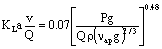(20)

Volumetric equations:(21)

The Recursive State and Parameter Estimator

State estimators are used to provide on-line values for variables measured indirectly or measured with a long delay time which describe the dynamic behavior of the system. These estimators are basically mathematical models of the system, solved on-line. They require some actual observed values that will be compared with the predicted ones, and the differences between these values are used to modify the estimation using the gain filter (Goodwin and Sin, 1984). Figure 1 shows the flow sheet of the recursive state estimator algorithm.

Figure 1:
Flow sheet of the recursive state estimator (Jazwinski, 1970).

State estimation is related to several mathematical techniques that use an internal process model associated with experimental measurement in order to calculate the process state (measured and unmeasured variables). The Kalman filter for linear systems uses process and measurement models, experimental measurement and statistical error information for the process in addition to observed variable noises in order to estimate the process state (Kalman, 1960; Kalman and Bucy, 1961). Therefore, those state variables not measured experimentally can be obtained and uncertainty is minimized for those that were measured. Such techniques were applied to nonlinear systems using the extended Kalman filter (Jazwinski, 1970; Goodwin and Sin, 1984; Gee and Ramirez, 1996).

The following equations present the dynamic model of the nonlinear process (Equation 22 and 23) and the Jacobian matrix with first-order terms, and the state and the measurement matrices of the discrete resulting model (Equations 24 to 27).(22)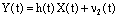(23)(24)(25)(26)(27)

The computational sequence of the extended Kalman filter is described as follows (Jazwinski, 1970; Montague et al., 1986):

(a) save the actual (k) state process:(b) calculate the predicted state:(c) calculate the covariance error:(d) calculate the filter gain:(e) calculate the state process (k+1):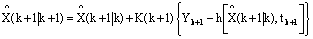(f) calculate the covariance error: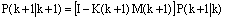(g) update the sample time k=k+1 and go back to step (a).

Frequently, the dynamic system model depends on certain parameters whose values are not accurately known. These parameters can be considered as outputs of the dynamic system, and they are added to vector U(t). Thus, the state vector of the system becomes.

The following equations represent the state vector (Equation 28), the observed vector (Equation 29), the simplified discrete model for penicillin production used as the internal model of the filter (Equation 30), the transition state matrix composed of the Jacobian matrix of the discrete model in respect to the state vector (Equation 31), the matrix composed of the Jacobian matrix of the observed vector in respect to the state vector (Equation 32), the covariance matrix of the system noise (Equation 33), the covariance matrix of the observed noise (Equation 34), the covariance matrix of the initial estimation errors (Equation 35) and the noise system coefficient matrix (Equation 36).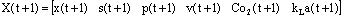(28)(29)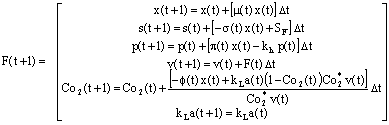(30)(31)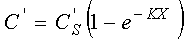(32)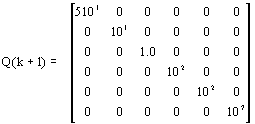(33)(34)(35)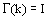(36)

(39)

The kinetic equations of the filter internal model, modified in relation to the complete model in such a way that a simplified metabolic structure is obtained, are shown as follows:(37)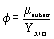(38)

It must be emphasized that the filter does not use a model for the estimation of the oxygen transfer coefficient because it is considered a parameter. In addition, some parameters of the complete model were modified in order to analyze the robustness of the recursive estimator.

RESULTS AND DISCUSSION

The cell, substrate, penicillin and dissolved oxygen concentrations, the fermenter volume and the oxygen volumetric coefficient were estimated by the extended Kalman filter using a simplified process model and actual observed values for amount of penicillin and concentration of dissolved oxygen with independent random Gaussian white noise obtained using a deterministic and nonstructured mathematical model.

The amount of penicillin and the concentration of dissolved oxygen were chosen as observed variables as the process is strict aerobic and a metabolite is produced. These variables are normally monitored in experimental works using an on-line electrode to measure the concentration of dissolved oxygen and an off-line analytical instrumental technique to measure the amount of penicillin. The latter analysis is rather time consuming in terms of computer time but the slow dynamics of the system and the sample period of the algorithm justify its use.

The performance of the filter was analyzed comparing the actual and the estimated state variable profiles. Three situations were defined such that the simulated variables obtained by the complete model would be as close to the experimental values as possible:

(a) errors were assumed to exist in the initial state values;

(b) random deviations of white noise were assumed to exist in the observed variables, whose values of standard deviation reproduce the analytical and sensor experimental errors;

(c) different parameters were assumed to exist in the complete process model in comparison with the internal filter model in order to reproduce the actual behavior of fermentative processes.

Table 1 presents the initial state variables, the parameters with deviations and the mean and standard deviations of the random error of the observed variables. The other parameters of the model are presented in the nomenclature section, and the optimal feed strategy is shown in Rodrigues and Maciel Filho (1996).

Table 1 - Initial state variables, parameters with deviation and mean and standard deviations of the random error of the observed variables.

The actual and the estimated state variable profiles are shown in Figures 2 to 7. The actual state variables were obtained using the complete process model and the estimated state variables were obtained using the extended Kalman filter. The estimator implemented was found to be robust because it reproduced the behavior of the process despite the initial state error and the random error of the observed variables.

Figure 2:
Actual cell concentration obtained using the complete process model and estimated cell concentration obtained using the extended Kalman filter.Figure 3: Actual substrate concentration obtained using the complete process model and estimated substrate concentration obtained using the extended Kalman filter.Figure 4: Actual penicillin concentration obtained using the complete process model and estimated penicillin concentration obtained using the extended Kalman filter.Figure 5: Actual dissolved oxygen concentration obtained using the complete process model and estimated dissolved oxygen concentration obtained using the extended Kalman filter.Figure 6: Actual volume obtained using the complete process model and estimated volume obtained using the extended Kalman filter.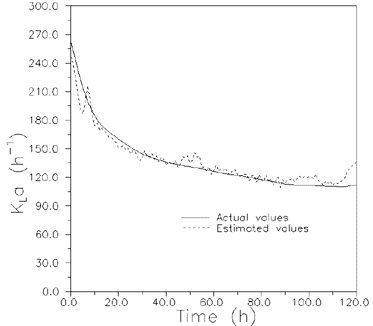Figure 7: Actual oxygen transfer coefficient obtained using the complete process model and estimated oxygen transfer coefficient obtained using the extended Kalman filter.

Although Figures 2 to 7 present variable profiles only for samples taken every hour, the estimator presented convergence problems when sampling periods were longer than 1 hour. However, this period is appropriate for experimental applications due to the slow dynamics of the system and the analysis time delay required for the determination of the amount of penicillin.

The estimator was found to be robust when the parameters of the complete model and the structure of the internal filter model were changed. However, the use of a suitable set of design parameters (Q, R and P0 vectors) was necessary.

The implementation of the extended Kalman filter presented two problems. First, it requires a reliable internal model that reproduces the behavior of the process. Second, it must calculate the transition state matrix composed of the Jacobian matrix of the discrete model in respect to the state vector through numerical techniques due to the nonlinear nature of the process. If these two points are not well calculated, the extended Kalman filter could fail since they are strictly related to the performance of the filter.

CONCLUSIONS

A recursive estimator of states (cell, substrate, product and dissolved oxygen concentrations, and the bioreactor volume) and one parameter (the oxygen transfer coefficient) using only two observed variables (the product amount and the concentration of dissolved oxygen) implemented in a fed-batch penicillin reactor based on the use of the extended Kalman filter was provided. It can be used for optimization and control purposes in a fermentative process which requires some state variables that are measured with a long delay time or unmeasured parameters.

The estimator was found to be robust reproducing the behavior of the process despite the initial state error, the random error of the observed variables, and the error of the parameters and the structure of the process model. However, the estimator presented convergence problems when sampling periods were longer than 1 hour. In addition, it requires a suitable set of design parameters, a reliable internal model that reproduces the behavior of the process, and a accurate estimation of the transition state matrix.

NOMENCLATURE

Penicillin Production Process Model

Co2 relative dissolved oxygen concentration (% of saturation) Co2* saturated concentration of dissolved oxygen (7.36 10-3 mmol/l) Cp product concentration (International Units/ml-1595 International Units = 1 mg Penicillin G) Cs substrate concentration (g/l) Cx cell concentration (g/l) Di impeller diameter (0.12 m) Em kinetic constant (1.0 g/l) Ep kinetic constant (1.0 g/l) F fed-batch flowrate (Rodrigues and Maciel Filho, 1996) (l/h) Fm endogenous fraction due maintenance (dimensionless) Fp endogenous fraction due production (dimensionless) g acceleration of gravity (9.81 m/s2) K consistency index (dynas.sn/cm2) K Metzners equation constant (11.5 dimensionless) Kh kinetic constant (0.01 h-1) Ki kinetic constant (0.1 g2/l2) KLa oxygen transfer coefficient (h-1) Kp kinetic constant (0.0001 g/l) Ks kinetic constant (0.06 l/g) Kx kinetic constant (0.006 g/g) Ms maintenance constant (0.029 g/g.l) n behavior index (0.39 dimensionless) NPo Power number (dimensionless) Nrem modified Reynolds number (dimensionless) Ns agitation speed (480 rps) p product amount (g) Pg power required for gasified fluid (W) Po power required for nongasified fluid (W) Q air flowrate (1.0 10-4 m3/s) Qo2 oxygen uptake rate (g/h) qo2 volumetric oxygen uptake rate (g/h.l) s substrate amount (g) SF substrate fed-batch concentration (321.3 g/l) t time (h) v volume of the fermentor (l) x cell amount (g) Yp/s product/substrate yield coefficient (g/g) Yx/o cell/oxygen yield coefficient (g/g) Yx/s cell/substrate yield coefficient (g/g)

Greek letters:

f specific oxygen uptake rate (g/g.h) g deformation rate (s-1) m specific growth rate (h-1) m ap apparent viscosity (Pa.s) m p highest specific product rate (h-1) m subst specific growth rate (h-1) m x highest specific growth rate (h-1) n ap apparent kinematic viscosity (m2/s) p specific production rate (h-1) r density (1.0 10 6 g/m3) s specific substrate rate (h-1)

Extended Kalman Filter Estimator

f dynamic model of the nonlinear process F process state vector h vector of the observed process variables I identity matrix k actual sample time K extended Kalman filter gain matrix M Jacobian matrix of the observed variables N parameter vector P covariance error matrix Q process noise covariance matrix R observed noise covariance matrix t time U input variables vector X process state vector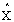estimated state vector Y

observed variables vector

Greek letters:

G system noise coefficients matrix f state transition matrix n 1 process noise vector n 2 observed noise vector

• Engasser, J.M., Bioreactor Engineering: The Design and Optimization of Reactors with Living Cells, Chemical Engineering Science, 43, 1739-1748 (1988).
• Gee, D.A. and Ramirez, W.F., On-Line State Estimation and Parameter Identification for Batch Fermentation, Biotechnology Progress, 12, 132-140 (1996).
• Goodwin, G.C. and Sin, K.S., Adaptive Filtering Prediction and Control. Prentice Hall, New York (1984).
• Jazwinski, A.H., Stochastic Processes and Filtering Theory. Academic Press, New York (1970).
• Kalman, R.E. and Bucy, R.S., New Results in Linear Filtering Prediction Theory, Trans. ASME, Ser. D: Journal Basic Engineering, 83, 95-108 (1961).
• Kalman, R.E., A New Approach to Linear Filtering and Prediction Problems, Trans. ASME, Ser. D: Journal Basic Engineering, 82, 35-45 (1960).
• Lee, S.C.; Hwang, Y.B.; Chang, H.N. and Chang, Y.K., Adaptive Control of Dissolved Oxygen Concentration in a Bioreactor, Biotechnology and Bioengineering, 37, 597-607 (1991).
• Montague, G.A.; Morris, A.J.; Wright, A.R.; Aynsley, M. and Ward, A., Modelling and Adaptive Control of Fed-Batch Penicillin Fermentation, Canadian Journal of Chemical Engineering, 64, 567-580 (1986).
• Rodrigues, J.A.D., Dinâmica e Estratégias de Otimizaçăo e Controle do Processo de Penicilina. Ph.D. diss., LOPCA/DPQ/FEQ/UNICAMP (1996).
• Rodrigues, J.A.D. and Maciel Filho, R., Optimal Feed Rates Strategies with Operating Constraints for the Penicillin Production Process, Chemical Engineering Science, 51, 2859-2864 (1996).
• Shimizu, K., An Overview on the Control System Design of Bioreactors, Advances in Biochemical Engineering Biotechnology, 50, 65-84 (1993).
• Stephanopoulos, G. and San, K.Y., Studies on On-Line Bioreactor Identification. I. Theory, Biotechnology and Bioengineering, 26, 1176-1188 (1984).
• *
To whom correspondence should be addressed

# Publication Dates

• Publication in this collection
23 Apr 1999
• Date of issue
Mar 1999

# History

• Received
14 Apr 1998
• Accepted
02 Feb 1999
Brazilian Society of Chemical Engineering Rua Líbero Badaró, 152 , 11. and., 01008-903 São Paulo SP Brazil, Tel.: +55 11 3107-8747, Fax.: +55 11 3104-4649, Fax: +55 11 3104-4649 - São Paulo - SP - Brazil
E-mail: rgiudici@usp.br